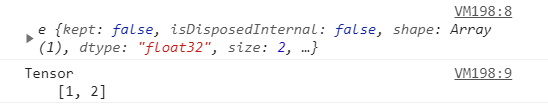Open in App
Not now

# Tensorflow.js tf.Tensor .toString() Method

• Last Updated : 17 May, 2021

Tensorflow.js is an open-source library developed by Google for running machine learning models and deep learning neural networks in the browser or node environment.

The tf.tensor.toString() method is used to log the tensor in the human-readable form if you console.log() just tf.tensor then it will log the whole tensor object but after using the .toString() method it will log the tensor’s value.

#### Syntax:

`tf.tensor.toString(verbose?)`

Parameter: This function accepts single parameter which are illustrated below:

• verbose: A boolean value. If it is true then the details of the tensor (dtype, rank, shape, values) will be returned otherwise only the tensor value will be returned. Although this is an optional parameter and by default it is false.

Return value: It returns the tensor value or tensor’s details (dtype, rank, shape, values) in string form.

Example 1: In this example, we are logging the tensor value before and after applying the toString() method.

## Javascript

 `// Importing the tensorflow.js library``import * as tf from ``"@tensorflow/tfjs"``  ` `// Creating the tensor``var` `val1 = tf.tensor([1, 2]);` `// Applying the toString() method``var` `val2 = val1.toString()` `// logging the tensor``console.log(val1)``console.log(val2)`

Output:Example 2: In this example, we apply the boolean parameters in the toString() method and see the results.

## Javascript

 `// Importing the tensorflow.js library``import * as tf from ``"@tensorflow/tfjs"``  ` `// Creating the tensor``var` `val = tf.tensor([1, 2]);` `// Applying the toString() method``var` `val1 = val.toString(``true``)``var` `val2 = val.toString(``false``)``// logging the tensor``console.log(val1)``console.log(val2)`

Output:

```Tensor
dtype: float32
rank: 1
shape: 
values:
[1, 2]
Tensor
[1, 2]```
My Personal Notes arrow_drop_up